# Thresholding and Calibration for Imbalanced Classification¶

Here we will go through an example of thresholding and calibration for an imbalanced dataset. The data we use in this example is click through rate (CTR) data. CTR is a ratio showing how often people who see your ad end up clicking it. CTR can be used to gauge how well your ads are performing. CTR is the number of clicks that your ad receives divided by the number of times your ad is shown:

• CTR = # of clicks on ads / # of views or impressions of ads

For example, if you had $5$ clicks and $100$ impressions, then your CTR would be $5\%$.

## Imports¶

In :
import warnings
import pandas as pd
import numpy as np
import matplotlib.pyplot as plt
import seaborn as sns
import matplotlib.cm as cm
from sklearn.preprocessing import StandardScaler
from sklearn.calibration import CalibratedClassifierCV, calibration_curve
from sklearn.model_selection import train_test_split, cross_val_score, KFold
from sklearn.metrics import (roc_curve, auc, roc_auc_score, plot_confusion_matrix, accuracy_score, f1_score,
precision_recall_curve, average_precision_score, brier_score_loss, recall_score,
confusion_matrix)
from sklearn.neural_network import MLPClassifier
from sklearn.linear_model import LogisticRegression
from xgboost import XGBClassifier
from sklearn.svm import SVC
from plot_utils import (plot_thresholds, plot_calibration_curve, comp_conf_matrix, plot_decision_surface)

In :
pd.set_option('display.max_columns', None)
sns.set(style="darkgrid", color_codes=True)
warnings.filterwarnings('ignore')

In :
def reduce_mem_usage(df, verbose=True):
numerics = ['int16', 'int32', 'int64', 'float16', 'float32', 'float64']
start_mem = df.memory_usage().sum() / 1024**2
for col in df.columns:
col_type = df[col].dtypes
if col_type in numerics:
c_min = df[col].min()
c_max = df[col].max()
if str(col_type)[:3] == 'int':
if c_min > np.iinfo(np.int8).min and c_max < np.iinfo(np.int8).max:
df[col] = df[col].astype(np.int8)
elif c_min > np.iinfo(np.int16).min and c_max < np.iinfo(np.int16).max:
df[col] = df[col].astype(np.int16)
elif c_min > np.iinfo(np.int32).min and c_max < np.iinfo(np.int32).max:
df[col] = df[col].astype(np.int32)
elif c_min > np.iinfo(np.int64).min and c_max < np.iinfo(np.int64).max:
df[col] = df[col].astype(np.int64)
else:
if c_min > np.finfo(np.float16).min and c_max < np.finfo(np.float16).max:
df[col] = df[col].astype(np.float16)
elif c_min > np.finfo(np.float32).min and c_max < np.finfo(np.float32).max:
df[col] = df[col].astype(np.float32)
else:
df[col] = df[col].astype(np.float64)

end_mem = df.memory_usage().sum() / 1024**2
if verbose: print('Mem. usage decreased to {:5.2f} Mb ({:.1f}% reduction)'.format(end_mem, 100 * (start_mem - end_mem) / start_mem))
return df

In :
df = pd.read_csv('prepro_ctr_data.csv').drop('Unnamed: 0', axis=1)
df = reduce_mem_usage(df)
print(len(df.index))
df = df.sample(n=550_000)

Mem. usage decreased to 92.51 Mb (49.5% reduction)
1000000

In :
df.info()

<class 'pandas.core.frame.DataFrame'>
Int64Index: 550000 entries, 611010 to 906874
Data columns (total 24 columns):
click               550000 non-null int8
C1                  550000 non-null int16
banner_pos          550000 non-null int8
site_id             550000 non-null int64
site_domain         550000 non-null int64
site_category       550000 non-null int64
app_id              550000 non-null int64
app_domain          550000 non-null int64
app_category        550000 non-null int64
device_id           550000 non-null int64
device_ip           550000 non-null int64
device_model        550000 non-null int64
device_type         550000 non-null int8
device_conn_type    550000 non-null int8
C14                 550000 non-null int16
C15                 550000 non-null int16
C16                 550000 non-null int16
C17                 550000 non-null int16
C18                 550000 non-null int8
C19                 550000 non-null int16
C20                 550000 non-null int32
C21                 550000 non-null int16
hour_of_day         550000 non-null int8
weekday             550000 non-null int8
dtypes: int16(7), int32(1), int64(9), int8(7)
memory usage: 55.1 MB


## Class Imbalance¶

CTR prediction is an example where classes are severely imbalanced and the distribution can often be modeled as a poisson random variable. In this dataset $1$ is for click $0$ is for no click.

In :
sns.countplot(x='click',data=df, palette='hls')
plt.show()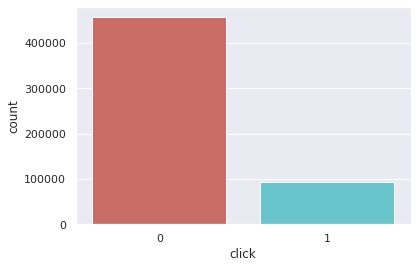In :
df.click.value_counts() / len(df.index)

Out:
0    0.830878
1    0.169122
Name: click, dtype: float64

## Fitting a Classifier With Imbalanced Data¶

Like many other learning algorithms in scikit-learn, some classifiers like LogisticRegression() or RandomForestClassifier()comes with a built-in method of handling imbalanced classes called class_weight. If we have highly imbalanced classes and have not addressed it during preprocessing, we have the option of using the class_weight parameter to weight the classes to help with having a balanced mix of each class. Specifically, the balanced argument will automatically weigh classes inversely proportional to their frequency giving most attention to the minority class:

$$w_j = \frac{n}{kn_j}$$

where $w_j$ is the weight to class $j,n$ is the number of observations, $n_j$ is the number of observations in class $j$, and $k$ is the total number of classes.

### XGBoost¶

When using XGBoost we can control the balance of positive and negative weights using the scale_pos_weight parameter. This parameter takes an integer and is typically the ratio of positive and negative intances in your target data:

• sum(negative instances) / sum(positive instances)
In :
X = df.drop('click', axis=1)
y = df.click

l_counts = y.value_counts().values
ratio = round(l_counts/l_counts)
ratio

Out:
5.0

## View Decision Regions¶

In :
from sklearn.decomposition import PCA
from sklearn.preprocessing import StandardScaler

classifiers = [XGBClassifier(n_estimators=1000, scale_pos_weight=ratio, n_jobs=-1, random_state=2),
MLPClassifier(activation='logistic', random_state=1, max_iter=200),
LogisticRegression(class_weight='balanced', random_state=1, n_jobs=-1)]

names = ['XGBoost', 'MLP', 'Logistic']

datasets = [df]

X0 = PCA(2).fit_transform(X.values)
X0 = StandardScaler().fit_transform(X0)
y0 = y.values

plot_decision_surface(X0, y0, classifiers, proba=True, size=[40,8], s=100)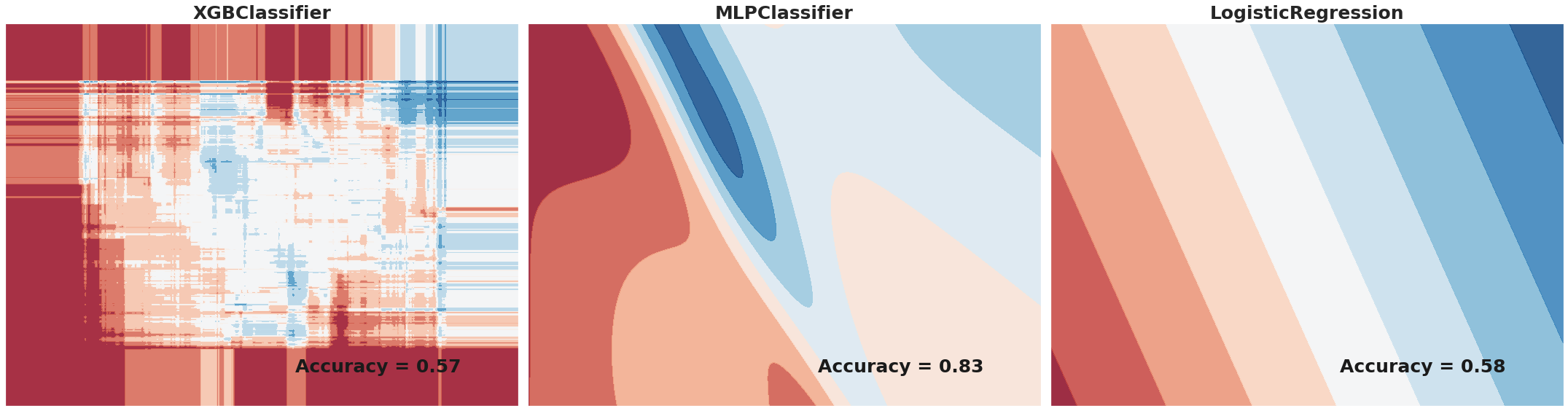Here we look at $3$ different classifiers XGBoost, MLP (neural network) and Logistic. Note these classifiers are fitted and scored using only the first $2$ principal components of the dataset for $2D$ visual representation. From the resulting plots you can see that XGBoost is capable of achieving a non-linear relationship. The MLP is also very adept at understanding non-linearity but, in this case there is no parameter to weigh predictions to the minority class or make a "cost-sensitive" neural network so, the resulting region is mostly the color of the majority class which is the cause of the much higher accuracy score (bottom right of plot). The logistic classifier prefers linearity in the data from the resulting decision regions.

## Scale and Split¶

In :
X = StandardScaler().fit_transform(X)
X_train, X_test, y_train, y_test = train_test_split(X, y, test_size=.4, random_state=42)


## Loop through classifiers will full dataset and score with auc¶

In :
scores = []
for name, clf in zip(names, classifiers):
clf.fit(X_train, y_train)
y_scores = clf.predict_proba(X_test)
score = roc_auc_score(y_test, y_scores[:, 1])
score = round(score,4)
print(f'{name}: roc_auc = {score}')
scores.append(score)

XGBoost: roc_auc = 0.7354
MLP: roc_auc = 0.7166
Logistic: roc_auc = 0.6325


# Threshold-Moving for Imbalanced Classification¶

Many machine learning algorithms are capable of predicting a probability or scoring of class membership, and this must be interpreted before it can be mapped to a class label. This is achieved by using a threshold, such as $0.5$ (often the default threshold), where all values equal or greater than the threshold are mapped to one class and all other values are mapped to another class.

• Prediction $< 0.5 =$ Class $0$
• Prediction $>= 0.5 =$ Class $1$

The problem is that the default threshold may not represent an optimal interpretation of the predicted probabilities. This might be the case for reasons, such as:

• The predicted probabilities are not calibrated, e.g. those predicted by an SVM or decision tree.
• The metric used to train the model is different from the metric used to evaluate a final model.
• The class distribution is severely imbalanced or skewed.
• The cost of one type of misclassification is more important than another type of misclassification.

## Optimal Threshold for ROC Curve¶

A ROC curve is a diagnostic plot that evaluates a set of probability predictions made by a model on a test dataset.

The false-positive rate is plotted on the x-axis and the true positive rate is plotted on the y-axis and the plot is referred to as the Receiver Operating Characteristic curve, or ROC curve. A diagonal line on the plot from the bottom-left to top-right indicates the “curve” for a no-skill classifier (predicts the majority class in all cases), and a point in the top left of the plot indicates a model with perfect skill.

The curve is useful to understand the trade-off in the true-positive rate and false-positive rate for different thresholds. The area under the ROC Curve, so-called ROC AUC, provides a single number to summarize the performance of a model in terms of its ROC Curve with a value between $0.5$ (no-skill) and $1.0$ (perfect skill).

We could locate the threshold with the optimal balance between false positive and true positive rates with the G-Mean. The Geometric Mean or G-Mean is a metric for imbalanced classification that, if optimized, will seek a balance between the sensitivity and the specificity.

• Sensitivity (recall or true positive rate) = TruePositive / (TruePositive + FalseNegative)
• Specificity (true negative rate) = TrueNegative / (FalsePositive + TrueNegative)
• G-Mean = sqrt(Sensitivity * Specificity)

### For our case predicting CTR¶

• If model predicts there is a click, then there is a bid for that impression which costs money
• If no click predicted, no bidding and hence no cost
• True positives (TP): money gained (impressions paid for that were clicked on).
• False positives (FP): money lost (impressions that were paid for, but not clicked).
• True negatives (TN): money saved (no click predicted so no impressions bought).
• False negatives (FN): money lost out on (no click predicted, but would have been actual click in reality).

## Optimal Threshold for Precision-Recall Curve¶

Unlike the ROC Curve, a precision-recall curve focuses on the performance of a classifier on the positive (minority class) only.

A precision-recall curve is calculated by creating accurate class labels for probability predictions across a set of thresholds and calculating the precision and recall for each threshold. A line plot is created for the thresholds in ascending order with recall on the x-axis and precision on the y-axis.

A no-skill model is represented by a horizontal line with a precision that is the ratio of positive examples in the dataset $0.01$ on our synthetic dataset. perfect skill classifier has full precision and recall with a dot in the top-right corner.

If we are interested in a threshold that results in the best balance of precision and recall, then this is the same as optimizing the F-measure that summarizes the harmonic mean of both measures.

• Precision = TruePositive / (TruePositive + FalsePositive)
• Recall = TruePositive / (TruePositive + FalseNegative)
• F-Measure = (2 Precision Recall) / (Precision + Recall)

### For our case predicting CTR¶

• Precision: proportion of clicks relative to total number of impressions
• Higher precision means higher ROI on ad spend
• Recall: the proportion of clicks gotten of all clicks available
• Higher recall means better targeting of relevant audience
In :
clf = XGBClassifier(n_estimators=1000, scale_pos_weight=ratio, n_jobs=-1, random_state=123)
clf.fit(X_train, y_train)
y_score = clf.predict_proba(X_test)
y_pred = clf.predict(X_test)

In :
plot_thresholds(X_test, y_test, y_score, name='XGBoost', fig_x=16, fig_y=5, sizes=[0.5, -160, -40])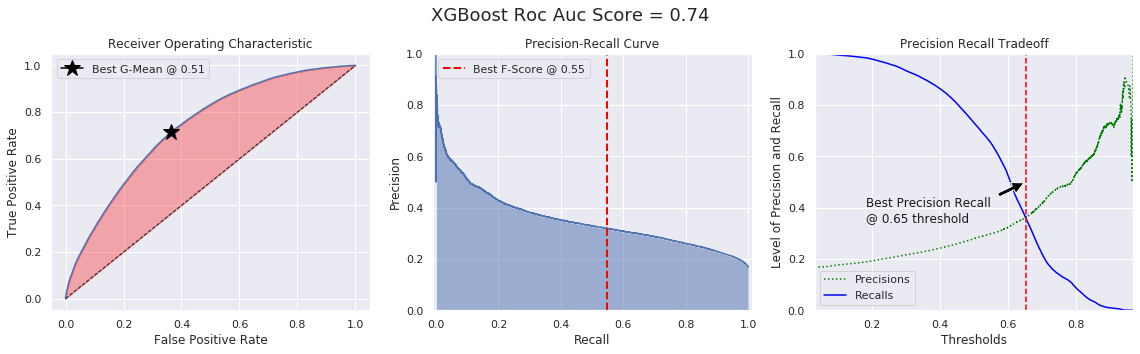In :
g_ts_pred = np.where(y_score[:, 1] > 0.51, 1, 0)
f1_ts_pred = np.where(y_score[:, 1] > 0.55, 1, 0)
prl_ts_pred = np.where(y_score[:, 1] > 0.65, 1, 0)

f1_ts_cm = np.around(confusion_matrix(y_test, f1_ts_pred, normalize='true'), decimals=3)
g_ts_cm = np.around(confusion_matrix(y_test, g_ts_pred, normalize='true'), decimals=3)
prl_ts_cm = np.around(confusion_matrix(y_test, prl_ts_pred, normalize='true'), decimals=3)
no_ts_cm = np.around(confusion_matrix(y_test, y_pred, normalize='true'), decimals=3)

comp_conf_matrix(mtx1=no_ts_cm, mtx2=f1_ts_cm, labels=['click', 'no click'],
titles=['No Threshold', 'F1 Thresholded'], sizes=[10, 4])

comp_conf_matrix(mtx1=prl_ts_cm, mtx2=g_ts_cm, labels=['click', 'no click'],
titles=['PRL Thresholded', 'G Thresholded'], sizes=[10, 4])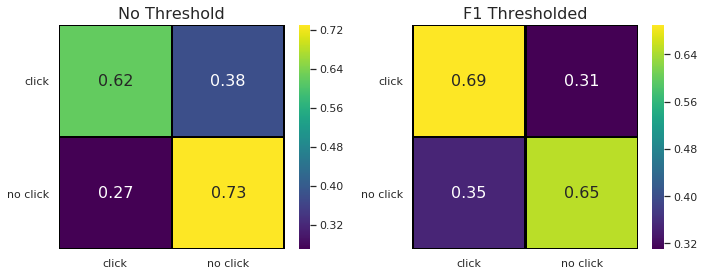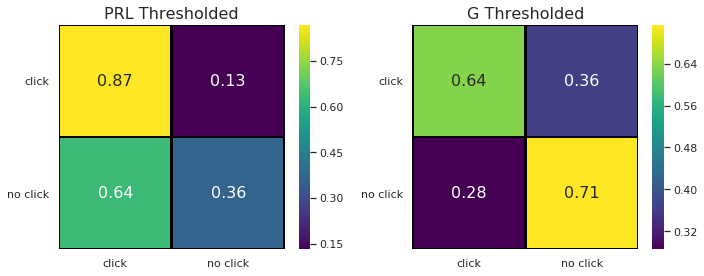The resulting confusion matrix from thresholding resulted in a much better balance in true positives and negatives. This is beneficial because we mostly are concerned with true positives money gained or impressions paid for that were clicked on.

# How and When to Use a Calibrated Classification Model¶

Predicted probabilities that match the expected distribution of probabilities for each class are referred to as calibrated. The problem is, not all machine learning models are capable of predicting calibrated probabilities.There are methods to diagnose how calibrated predicted probabilities are and methods to better calibrate the predicted probabilities with the observed distribution of each class. Often, this can lead to better quality predictions, depending on how the skill of the model is evaluated.

Although a model may be able to predict probabilities, the distribution and behavior of the probabilities may not match the expected distribution of observed probabilities in the training data. This is especially common with complex nonlinear machine learning algorithms that do not directly make probabilistic predictions and instead use approximations.

The distribution of the probabilities can be adjusted to better match the expected distribution observed in the data. This adjustment is referred to as calibration, as in the calibration of the model or the calibration of the distribution of class probabilities.

## Reliability Diagrams (Calibration Curves)¶

A reliability diagram is a line plot of the relative frequency of what was observed (y-axis) versus the predicted probability frequency (x-axis).

Specifically, the predicted probabilities are divided up into a fixed number of buckets along the x-axis. The number of events (class=1) are then counted for each bin (e.g. the relative observed frequency). Finally, the counts are normalized. The results are then plotted as a line plot.

These plots are commonly referred to as ‘calibration‘ plots or curves as they summarize how well the forecast probabilities are calibrated. The better calibrated or more reliable a forecast, the closer the points will appear along the main diagonal from the bottom left to the top right of the plot.

The position of the points or the curve relative to the diagonal can help to interpret the probabilities; for example:

• Below the diagonal: The model has over-forecast; the probabilities are too large.
• Above the diagonal: The model has under-forecast; the probabilities are too small.

Probabilities are continuous, so we expect some deviation from the line, often shown as an S-shaped curve showing tendencies of over-forecasting low probabilities and under-forecasting high probabilities.

## Probability Calibration¶

Some algorithms are fit in such a way that their predicted probabilities are already calibrated like a logistic regression model where the overall average predicted probability is equal to the average of the response.

Other algorithms do not directly produce predictions of probabilities, and instead a prediction of probabilities must be approximated. Some examples include neural networks, support vector machines, and decision trees.

Calibration of prediction probabilities is a rescaling operation that is applied after the predictions have been made by a predictive model. There are two popular approaches to calibrating probabilities; they are the Platt Scaling and Isotonic Regression.

Platt Scaling is simpler and is suitable for reliability diagrams with the S-shape. Isotonic Regression is more complex, requires a lot more data (otherwise it may overfit), but can support reliability diagrams with different shapes (is nonparametric).

Keep in mind better calibrated probabilities may or may not lead to better class-based or probability-based predictions. It really depends on the specific metric used to evaluate predictions. In fact, some empirical results suggest that the algorithms that can benefit the more from calibrating predicted probabilities include SVMs, bagged decision trees, and random forests.

In :
plot_calibration_curve(y, X_train, X_test, y_train, y_test, clf, "XGBoost", fig_idx=1, n_cv=5,
fig_x=10, fig_y=10, imb=True, C=1)

Logistic:
Brier: 0.235
Precision: 0.220
Recall: 0.610
F1: 0.324

XGBoost:
Brier: 0.212
Precision: 0.280
Recall: 0.730
F1: 0.405

XGBoost + Isotonic:
Brier: 0.126
Precision: 0.573
Recall: 0.065
F1: 0.116

XGBoost + Sigmoid:
Brier: 0.126
Precision: 0.591
Recall: 0.045
F1: 0.083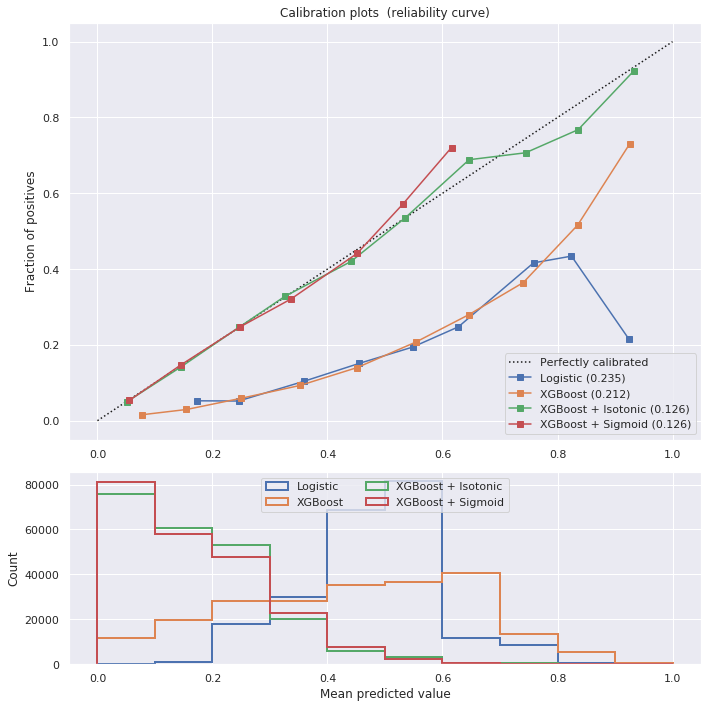From the resulting reliability diagram it looks like sigmoid (platts method) increases our precision the most.

## Fit and Evaluate Calibrated Classifier¶

In :
sigmoid = CalibratedClassifierCV(clf, cv=5, method='sigmoid')
sigmoid.fit(X_train, y_train)
y_score = sigmoid.predict_proba(X_test)
y_pred = sigmoid.predict(X_test)

In :
plot_thresholds(X_test, y_test, y_score, name='XGBoost', fig_x=16, fig_y=5, sizes=[0.5, 25, -40])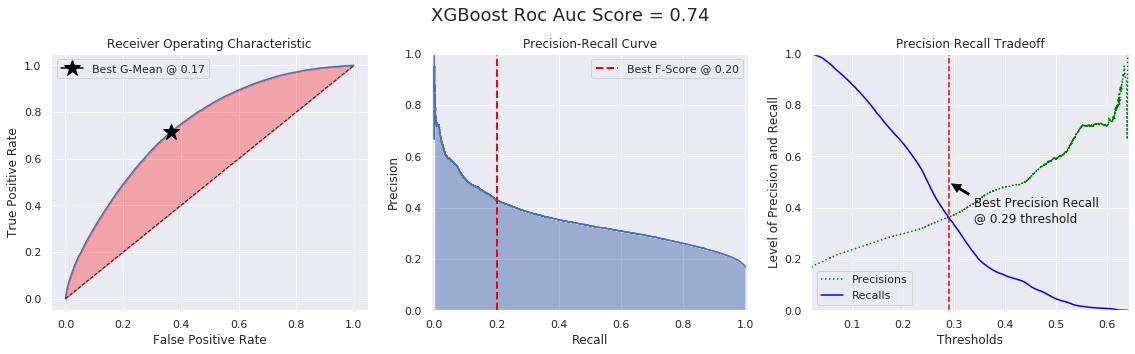In :
g_cal_ts_pred = np.where(y_score[:, 1] > 0.17, 1, 0)
f1_cal_ts_pred = np.where(y_score[:, 1] > 0.20, 1, 0)
prl_cal_ts_pred = np.where(y_score[:, 1] > 0.29, 1, 0)

g_cal_ts_cm = np.around(confusion_matrix(y_test, g_cal_ts_pred, normalize='true'), decimals=3)
f1_cal_ts_cm = np.around(confusion_matrix(y_test, f1_cal_ts_pred, normalize='true'), decimals=3)
prl_cal_ts_cm = np.around(confusion_matrix(y_test, prl_cal_ts_pred, normalize='true'), decimals=3)
cal_no_ts_cm = np.around(confusion_matrix(y_test, y_pred, normalize='true'), decimals=3)

comp_conf_matrix(mtx1=cal_no_ts_cm, mtx2=f1_cal_ts_cm, labels=['click', 'no click'],
titles=['Cal No Threshold', 'Cal F1 Thresholded'], sizes=[10, 4])

comp_conf_matrix(mtx1=prl_cal_ts_cm, mtx2=g_cal_ts_cm, labels=['click', 'no click'],
titles=['Cal PRL Thresholded', 'Cal G Thresholded'], sizes=[10, 4])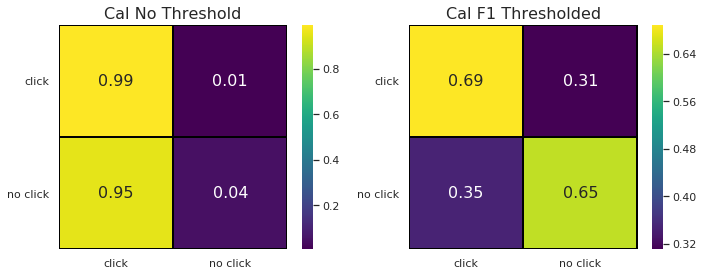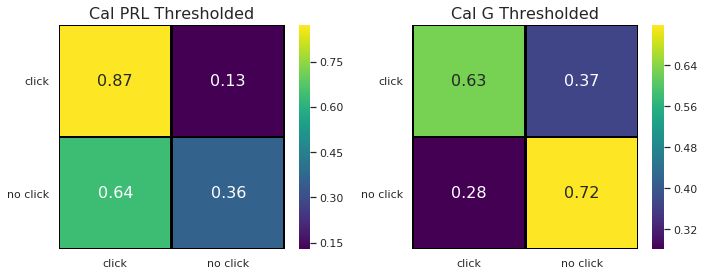The resulting confusion matrix from thresholding and calibrating looks to be beneficial. Once again we mostly are concerned with true positives or impressions paid for that were clicked on (ad ROI) and there was a valuable increase in true positives.

### Precision, ROI, and AUC¶

The return on investment (ROI) for ad spend can be categorized using the four outcomes from a confusion matrix. This quantity is defined as the ratio between the total return and the total cost. If this quantity is greater than $1$, it indicates the total return was greater than the total cost and vice versa. In this exercise, we will compute a sample ROI assuming a fixed r, the return on a click per number of impressions, and cost, the cost per number of impressions.

In :
def compare_roi(matrs=None, titles=None):

for i in range(len(matrs)):
# Compute confusion matrix and get four categories
tn, fp, fn, tp = matrs[i].ravel()

# Calculate total return, total spent, and ROI
r = 0.2
cost = 0.05

total_return = tp * r
total_cost = (tp + fp) * cost
roi = total_return / total_cost

print(f"{titles[i]} Total return: %s, Total cost: %s, ROI: %s" %(round(total_return,2),
round(total_cost,2), round(roi,2)))

In :
compare_roi(matrs=[no_ts_cm, f1_ts_cm, prl_ts_cm, g_ts_cm, f1_cal_ts_cm, prl_cal_ts_cm, g_cal_ts_cm],
titles=['No Threshold', 'F1 Thresholded', 'PRL Thresholded', 'G Thresholded',
'Cal F1 Thresholded', 'Cal PRL Thresholded', 'Cal G Thresholded'])

No Threshold Total return: 0.15, Total cost: 0.06, ROI: 2.63
F1 Thresholded Total return: 0.13, Total cost: 0.05, ROI: 2.71
PRL Thresholded Total return: 0.07, Total cost: 0.02, ROI: 2.94
G Thresholded Total return: 0.14, Total cost: 0.05, ROI: 2.65
Cal F1 Thresholded Total return: 0.13, Total cost: 0.05, ROI: 2.71
Cal PRL Thresholded Total return: 0.07, Total cost: 0.02, ROI: 2.96
Cal G Thresholded Total return: 0.14, Total cost: 0.05, ROI: 2.64


The calibrated precision recall line thresholded model has the greatest ROI

In [ ]: